# McKinsey - Hack the crash

Published: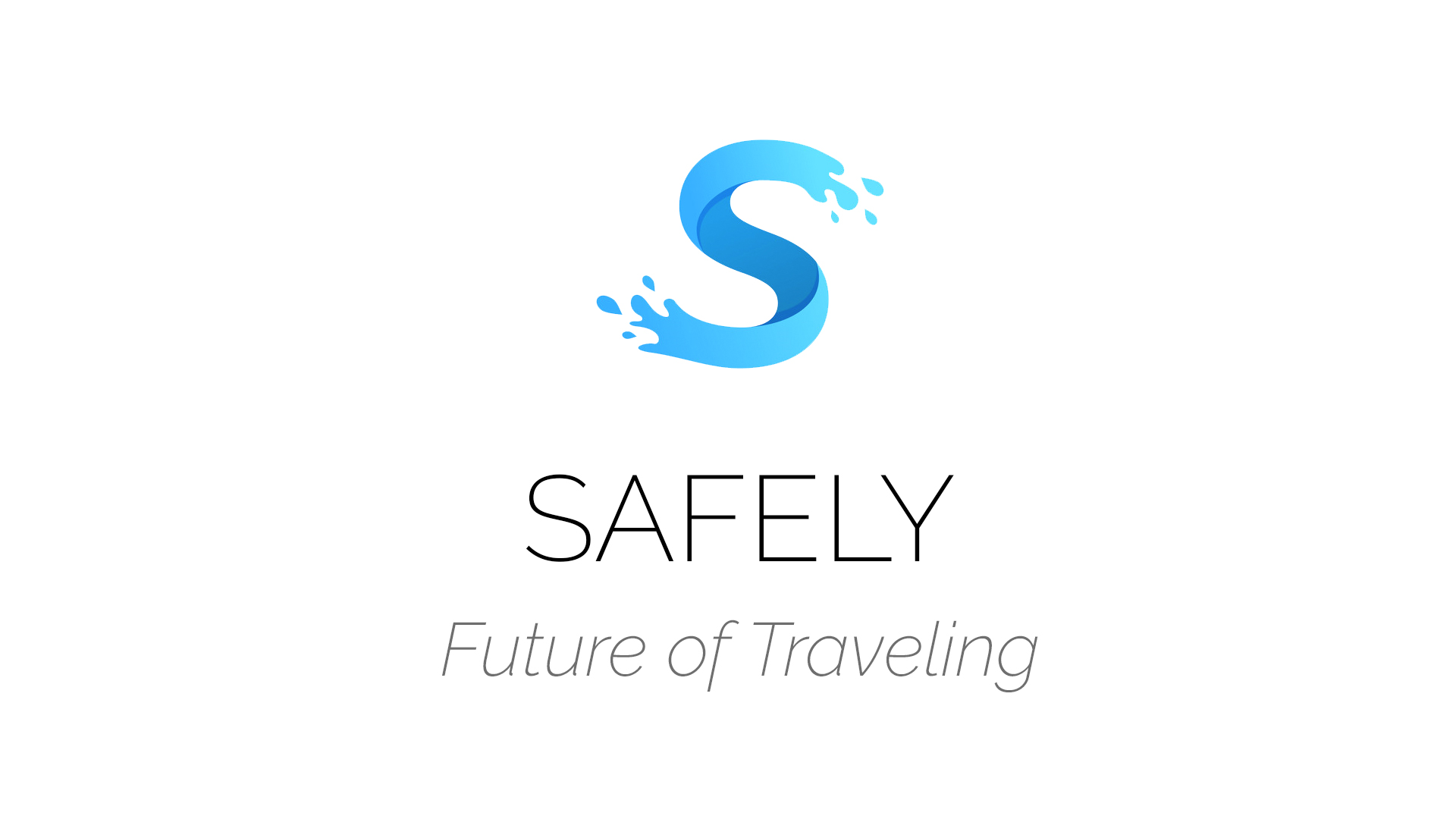## Data Preprocessing

• Correlation - measured between features and target value allowed sometimes to decide if drop column.

def check_corelation(csv, col_1, col_2):
df_corr = pd.DataFrame()
df_corr[col_1] = csv[col_1].astype('category').cat.codes
df_corr[col_2] = csv[col_2]
df_corr = df_corr.dropna()
print(df_corr.corr())

• Factorization

def factorize(csv, col_name):
dummy = pd.get_dummies(csv[col_name])
dummy.columns = [col_name + " " + str(x) for x in dummy.columns]
csv = csv.drop(col_name, axis=1)
csv = pd.concat([csv, dummy], axis=1)
return csv

• Feature Extraction - some features were extracted manually

time = pd.DatetimeIndex(csv["time"])
time = time.hour * 60 + time.minute
time = pd.DataFrame(time)
time[time >= 1080] = "evening"
time[time >= 720] = "midday"
time[time >= 360] = "morning"
time[time >= 0] = "night"
csv["day_time"] = time
csv = factorize(csv, "day_time")
csv = csv.drop("time", axis=1)

• Standarization

logregPipe = Pipeline([('scaler', StandardScaler()),('logreg', LogisticRegression())])
x_train, y_train = logregPipe.fit_transform(x_train, y_train)


## Feature Selection

• R² Denoisser - I’ve used two regerssors inside denoiser: Decision Tree Regressior and K-Neares Neighbours Regressor

R² Score Denoisser
Regressor: DecisionTreeRegressor
Number of Selected Features: 71
R² Score Denoisser
Regressor: KNeighborsRegressor
Number of Selected Features: 68

• Boruta Selection - resulted in 16 features.

Boruta gives closer look at selected features. The most important were Light and Weather Condition.

BorutaPy finished running.
Iteration:  20 / 100
Confirmed:  16
Tentative:  0
Rejected:   87
Number of Selected Features: 16

• PCA - I’ve also tried Principal Component Analysis to select the most informative features.

  def pca_decomp(X_train, Y_train):
X_train = StandardScaler().fit_transform(X_train)
pca = PCA(n_components=0.9, svd_solver='full', random_state=0)
X_train = pca.fit_transform(X_train)
x_train, x_test, y_train, y_test = train_test_split(X_train,Y_train,test_size=0.3)
return (x_train, x_test, y_train, y_test)


## Data Analysis

Plotting distributions also helps to realize if selected features behave properly just before training.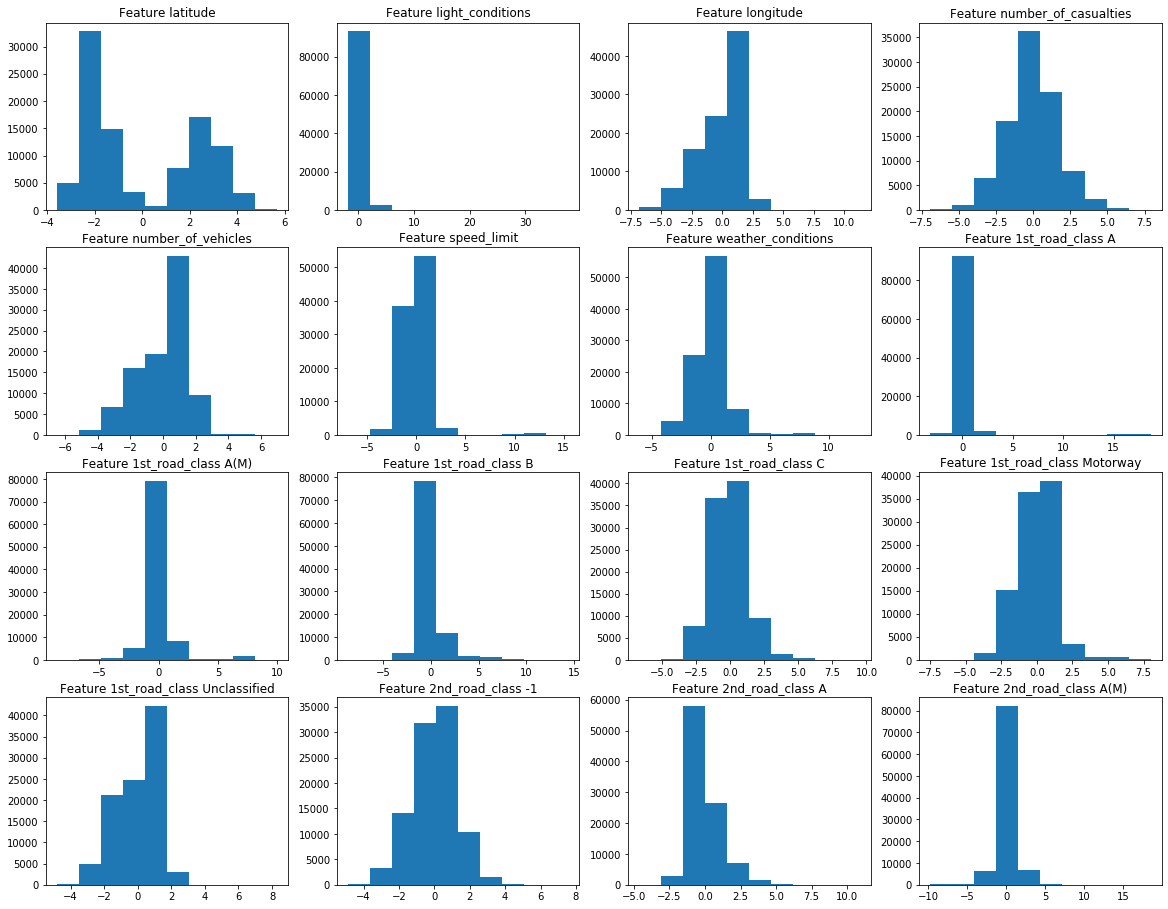## Machine Learning Pipeline

I’ve used custom pipeline strategy to train and test 5 different ML algorithms. I’ll describe it on example of Decision Tree Classifier.

• Pipeline - I’ve used it to perform automated Scaling

dectreePipe = Pipeline([('scaler', StandardScaler()), ('dectree', DecisionTreeClassifier())])
dectreeParam = {
'dectree__criterion': ['entropy', 'gini'],
'dectree__class_weight': ['balanced', None],
'dectree__max_depth': range(1, 7),
}

• GridSearchCV - I’ve used Grid Search with Cross Validation to select the best model

dectreeGS = GridSearchCV(dectreePipe, param_grid=dectreeParam, cv=5,
scoring='f1').fit(x_train, y_train)
mean_test_score = dectreeGS.cv_results_["mean_test_score"]
std_test_score = dectreeGS.cv_results_["std_test_score"]
plt.figure(figsize=(4, 2))
plt.errorbar(np.arange(mean_test_score.shape), mean_test_score,
std_test_score, fmt='ok')
selected parameter
class_weight           balanced
criterion               entropy
max_depth                     3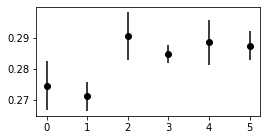• Confusion matrix is always a best way to visualize the prediction

Predicted 0Predicted 1
Actual 01407320092
Actual 124634652

## Model Selection

• Logistic Regression - unfortunately GridSearch won’t find any parameters that would give satisfiable solution. ROC looks almost flat, we will move forward.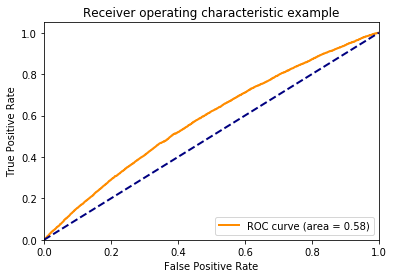• K-Neighbors Classifier - results were better than LogReg but still we need more.

knnPipe = Pipeline([
('scaler', StandardScaler()),
('knn', KNeighborsClassifier(n_jobs=-1)),
])
knnParam = {
'knn__n_neighbors': range(1, 12),
'knn__weights': ['uniform', 'distance'],
}
_ = knnPipe.fit(x_train, y_train)
f1_score on the train set:  0.066
f1_score on the test set:  0.028

• Decision Tree Classifier - this one give me the best results . Score below is after training on 10% part of training data, later there will be performed final training.

f1_score on the train set:  0.297
f1_score on the test set: 0.292
selected parameter
class_weight           balanced
criterion               entropy
max_depth                     3

• Random Forest - It was very hard to find proper parameters for that algorithm.

                selected parameter
bootstrap                 True
max_depth                 20
max_features              auto
min_samples_leaf          1
min_samples_split         2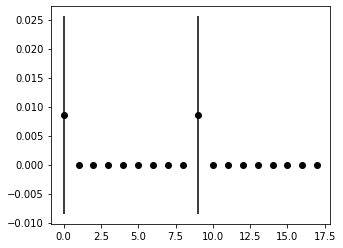• XGBoost - even though it’s one of most popular ML algorithm it looses this time with overfitting.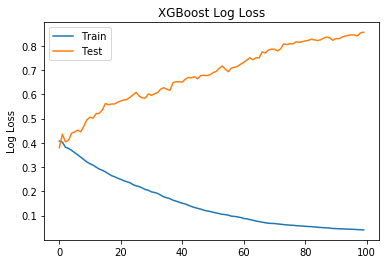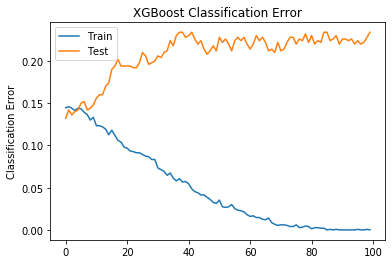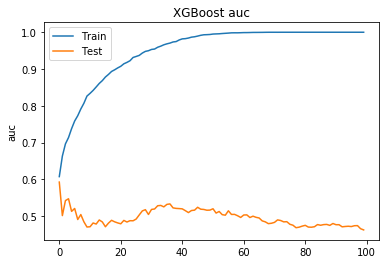• Quick Summary of parameters Grid Search.

• Logistic Regression f1_score on the train set: 0.0265 f1_score on the test set: 0.0
• K-Neighbors Classifier f1_score on the train set: 1.0 f1_score on the test set: 0.102
• Decision Tree Classifier f1_score on the train set: 0.291 f1_score on the test set: 0.279
• RandomForestClassifier f1_score on the train set 0.969 f1_score on the test set 0.0
• XGBoost f1_score on the train set 1.0 f1_score on the test set 0.048

We choose Decision Tree Classifier as our algorithm.

## Model Selection - Part II - choosing features

• PCA 68 CV f1 on the train set: 31.3 CV f1 on the test set: 30.8
• Denoisser KNR 68 CV f1 on the train set: 31.04 CV f1 on the test set: 30.75
• Denoisser DTR 71 CV f1 on the train set: 30.81 CV f1 on the test set: 31.2
• Boruta 16 CV f1 on the train set: 34.59 CV f1 on the test set: 33.8
• All features 103 CV f1 on the train set: 34.19 CV f1 on the test set: 34.17

As a result none of our feature selection technique performed better than whole features set.

## Lesson for future projects

• Try to use PCA and other dimension reducing techniques as LDA or QDA instead of feature selection.
• Know your algorithm parameters to properly GridSearch over them.
• Feature processing may be very time consuming use functions and generalize your tasks.

## Summary

The best algorithm for predicting damage inflicted in traffic accidents is

DecisionTreeClassifier(criterion='entropy', class_weight='balanced', max_depth=5)


working on all features and whole set gained F1 Score = 34.17

Mateusz Dorobek, Piotr Podbielski, Aitor Mato, Jaume Mora Viñes - Team Safely - HACK UPC 2019Tags: